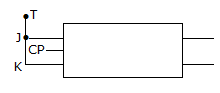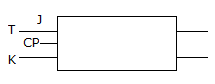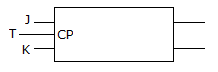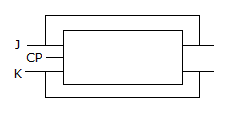# Electronics and Communication Engineering - Digital Electronics

21.

DeMorgan's second theorem is

 A. A . A = 0 B. A = A C. A + B = A . B D. AB = A + B

Explanation:

No answer description available for this question. Let us discuss.

22.

In a D latch

 A. a high D sets the latch and low D resets it B. a low D sets the latch and high D resets it C. race can occur D. none of the above

Explanation:

No answer description available for this question. Let us discuss.

23.

In floating point representation, mantissa is expressed in

 A. excess 3 notation B. gray code C. excess 32 notation D. 2's complement

Explanation:

No answer description available for this question. Let us discuss.

24.

Which of the circuits in the given figure that converts JK flip flop to T flip flop?

 A.B.C.D.Explanation:

No answer description available for this question. Let us discuss.

25.

In the generic microprocessor

 A. instruction cycle time period is shorter than machine cycle time period B. machine cycle time period is shorter than instruction cycle time period C. instruction cycle time period is exactly half of machine cycle time period D. instruction cycle time period is exactly equal to machine cycle time period